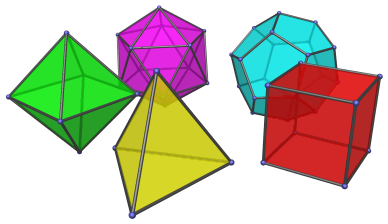# The Regular Polyhedra

A basic understanding of 3D polyhedra is important for understanding 4D polychora, because the cells of 4D polychora are 3D polyhedra. In particular, the cells of the regular polychora are regular polyhedra.

A polyhedron is said to be regular if its faces and vertex figures (the figure that results when a vertex is cut off) are regular polygons. There are five possible convex regular polyhedra in 3D. These are also known as the Platonic solids.• The regular tetrahedron, shown above in yellow, is bounded by 4 equilateral triangles. It is self-dual: the convex hull of the centers of its faces is also a regular tetrahedron.

• The cube, shown above in red, is bounded by 6 squares. It is dual to the octahedron. The convex hull of the centers of its faces is the octahedron.

• The octahedron, shown above in green, is bounded by 8 equilateral triangles. It is dual to the cube.

• The dodecahedron, shown above in cyan, is bounded by 12 regular pentagons, and is dual to the icosahedron.

• The icosahedron, shown above in magenta, is bounded by 20 equilateral triangles, and is dual to the dodecahedron.

## Constructions

All 5 Platonic solids can be constructed from the cube.

### The Cube

The cube itself is easily constructed by taking all permutations of sign of the point:

• (1, 1, 1)

These coordinates yield a cube with edge length 2.

### The Tetrahedron

Having constructed the cube, we can color each of its 8 vertices red or green, such that every edge connects a red vertex to a green vertex. For example, we can assign red to vertices which have an even number (including 0) of negative signs, and green to vertices with an odd number of negative signs. This forms two groups of vertices: 4 red vertices and 4 green vertices. If we throw away the green vertices, the remaining 4 red vertices form a tetrahedron. This operation is called alternation, and can be applied to any n-dimensional hypercube. Thus, the tetrahedron's coordinates are found to be (1,1,1) with all even changes of sign, that is:

• (1, 1, 1)
• (1, -1, -1)
• (-1, 1, -1)
• (-1, -1, 1)

### The Octahedron

We could have thrown away the red vertices of the cube and kept the green ones instead, and we would obtain another tetrahedron which is in dual orientation to the first one. This dual tetrahedron's vertices are the vertices of the cube with an odd number of negative signs. The intersection of these two tetrahedra gives us an octahedron. Since the edges of the two dual tetrahedra bisect each other, the vertices of the octahedron must lie at their midpoints, which are all permutations of coordinates and changes of sign of:

• (1, 0, 0)

### The Icosahedron

Now, the six vertices of the octahedron can be grouped into three pairs of opposites; we can color them red, green, and blue, respectively. Decide on a cyclic ordering of colors, say, red-green-blue. Since each face of the octahedron will have three different-colored vertices, we can assign a direction to each edge based on our cyclic ordering: for example, we can say that red goes to green, green goes to blue, and blue goes to red. Such an assignment of edge direction is called a “coherent indexing”, which allows us to consistently divide each edge in some ratio A:B according to its direction. If we divide each of the octahedron's edges in the Golden Ratio, φ=(1+√5)/2, we obtain the 12 vertices of the icosahedron as all even permutations of coordinates of (0,±φ−1,±1), or if we scale the icosahedron by a factor of φ, all even permutations of:

• (0, ±1, ±φ)

### The Dodecahedron

Finally, by taking the dual of the icosahedron, we obtain the dodecahedron. Computing the midpoints of the icosahedron's faces yields the coordinates of the dodecahedron, which, scaled by a factor of 3φ2, are all of changes of sign of:

• (1, 1, 1)

as well as all even permutations of coordinates and all changes of sign of:

• (0, φ−1, φ)

Last updated 06 Feb 2018.